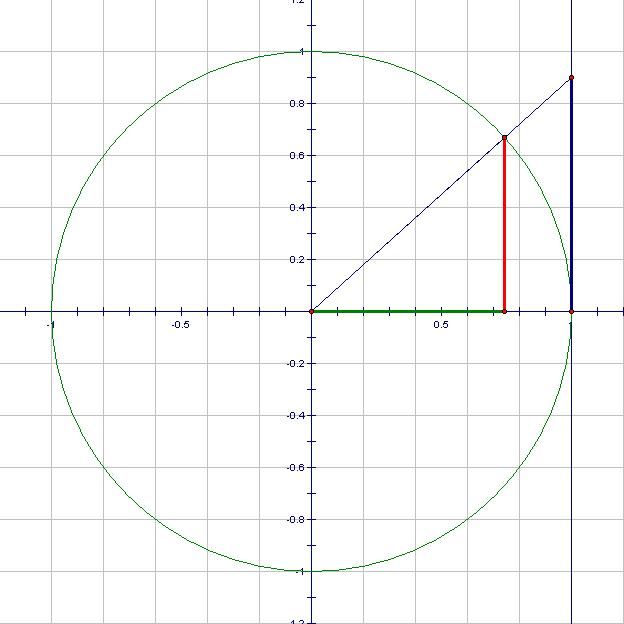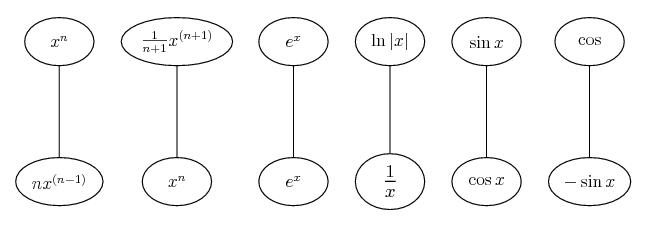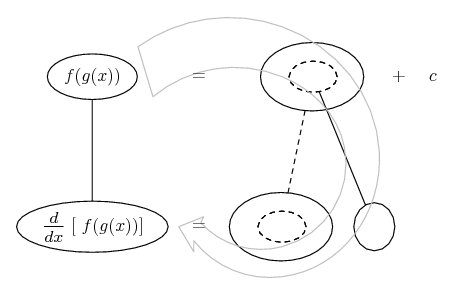# Thread: Trig identity and ln derivatives driving me nuts!

1. ## Trig identity and ln derivatives driving me nuts!

I'm working my way through all the Khan Academy topics in the practice section and I've come as far as 'special derivatives' and 'chain rule'. The special derivatives consist of identifying the derivatives of sin, cos, tan, ln and e. I have memorized the derivative of these functions but I do not understand why they work (cannot find a good proof).

In the chain rule and beyond, almost every problem has these special derivatives in them and I am finding it really hard to find good information containing proofs for these.

For example I memorized that the derivative of ln is 1/x and also I memorized that the derivative of tan(x) is sec^2(x). Then I got a problem which asked me to derive ln*tan(x) which somehow started going in the direction of 1/tan(x) and I'm clueless.

Please help me find some good stuff on this as I've been stuck here for a long time and I was progressing extremely fast in math before this!

2. ## Re: Trig identity and ln derivatives driving me nuts!Originally Posted by PazeI'm working my way through all the Khan Academy topics in the practice section and I've come as far as 'special derivatives' and 'chain rule'. The special derivatives consist of identifying the derivatives of sin, cos, tan, ln and e. I have memorized the derivative of these functions but I do not understand why they work (cannot find a good proof).

In the chain rule and beyond, almost every problem has these special derivatives in them and I am finding it really hard to find good information containing proofs for these.

For example I memorized that the derivative of ln is 1/x and also I memorized that the derivative of tan(x) is sec^2(x). Then I got a problem which asked me to derive ln*tan(x) which somehow started going in the direction of 1/tan(x) and I'm clueless.

Please help me find some good stuff on this as I've been stuck here for a long time and I was progressing extremely fast in math before this!
The proof that \displaystyle \displaystyle \begin{align*} \frac{d}{dx}\left[ \sin{(x)} \right] = \cos{(x)} \end{align*} requires knowing the standard limits \displaystyle \displaystyle \begin{align*} \lim_{h \to 0}\frac{\sin{(h)}}{h} = 1 \end{align*} and \displaystyle \displaystyle \begin{align*} \lim_{h \to 0}\frac{\cos{(h)} - 1}{h} = 0 \end{align*}. Proof:

Let \displaystyle \displaystyle \begin{align*} f(x) = \sin{(x)} \end{align*}, then \displaystyle \displaystyle \begin{align*} f(x + h) = \sin{(x + h)} = \sin{(x)}\cos{(h)} + \cos{(x)}\sin{(h)} \end{align*}, and so

\displaystyle \displaystyle \begin{align*} f'(x) &= \lim_{h \to 0}\frac{f(x + h) - f(x)}{h} \\ &= \lim_{h \to 0}\frac{\sin{(x)}\cos{(h)} + \cos{(x)}\sin{(h)} - \sin{(x)}}{h} \\ &= \lim_{h \to 0}\frac{\sin{(x)}\cos{(h)} - \sin{(x)}}{h} + \lim_{h \to 0}\frac{\cos{(x)}\sin{(h)}}{h} \\ &= \sin{(x)}\lim_{h \to 0}\left[ \frac{\cos{(x)} - 1}{h} \right] + \cos{(x)} \lim_{h \to 0}\frac{\sin{(h)}}{h} \\ &= \sin{(x)} \cdot 0 + \cos{(x)} \cdot 1 \\ &= \cos{(x)} \end{align*}

Q.E.D.

Proof that \displaystyle \displaystyle \begin{align*} \frac{d}{dx} \left[ \cos{(x)} \right] = -\sin{(x)} \end{align*}:

\displaystyle \displaystyle \begin{align*} y &= \cos{(x)} \\ &= \sin{\left( \frac{\pi}{2} - x \right)} \end{align*}

Now let \displaystyle \displaystyle \begin{align*} u = \frac{\pi}{2} - x \end{align*} so that \displaystyle \displaystyle \begin{align*} y = \sin{(u)} \end{align*}.

Then \displaystyle \displaystyle \begin{align*} \frac{du}{dx} = -1 \end{align*} and

\displaystyle \displaystyle \begin{align*} \frac{dy}{du} &= \cos{(u)} \\ &= \cos{\left( \frac{\pi}{2} - x \right)} \\ &= \sin{(x)} \end{align*}

And thus \displaystyle \displaystyle \begin{align*} \frac{dy}{dx} = -1 \cdot \sin{(x)} = -\sin{(x)} \end{align*}.

Q.E.D.

Proof that \displaystyle \displaystyle \begin{align*} \frac{d}{dx} \left[ \tan{(x)} \right] = \sec^2{(x)} \end{align*}:

\displaystyle \displaystyle \begin{align*} y &= \tan{(x)} \\ &= \frac{\sin{(x)}}{\cos{(x)}} \\ \frac{dy}{dx} &= \frac{\cos{(x)}\frac{d}{dx}\left[ \sin{(x)} \right] - \sin{(x)} \frac{d}{dx} \left[ \cos{(x)} \right]}{\left[ \cos{(x)} \right]^2} \\ &= \frac{\cos{(x)}\cos{(x)} - \sin{(x)}\left[ -\sin{(x)} \right] }{\cos^2{(x)}} \\ &= \frac{\cos^2{(x)} + \sin^2{(x)}}{\cos^2{(x)}} \\ &= \frac{1}{\cos^2{(x)}} \\ &= \sec^2{(x)} \end{align*}

We have \displaystyle \displaystyle \begin{align*} \frac{d}{dx}\left( e^x \right) = e^x \end{align*} by definition. There's not much of a proof we can do here, except to set up \displaystyle \displaystyle \begin{align*} f(x) = a^x \end{align*}, get a limit expression for \displaystyle \displaystyle \begin{align*} f'(x) \end{align*}, and use this to evaluate the value of \displaystyle \displaystyle \begin{align*} a \end{align*} so that \displaystyle \displaystyle \begin{align*} f(x) = f'(x) \end{align*}.

The proof that \displaystyle \displaystyle \begin{align*} \frac{d}{dx} \left[ \ln{(x)} \right] = \frac{1}{x} \end{align*} requires knowing that \displaystyle \displaystyle \begin{align*} \frac{dy}{dx} = \frac{1}{\frac{dx}{dy}} \end{align*}.

\displaystyle \displaystyle \begin{align*} y &= \ln{(x)} \\ x &= e^y \\ \frac{dx}{dy} &= e^y \\ \frac{1}{\frac{dx}{dy}} &= \frac{1}{e^y} \\ \frac{dy}{dx} &= \frac{1}{x} \end{align*}

Q.E.D.

All derivatives of combinations of these special functions can be evaluated using your standard sum, difference, product, quotient and chain rules.

3. ## Re: Trig identity and ln derivatives driving me nuts!

Wow, thanks a lot. I'm going to go through these when I wake up tomorrow!

Again, thanks a lot!

4. ## Re: Trig identity and ln derivatives driving me nuts!

After looking over these I am definitely starting to see a pattern, however I do not possess proper understanding for the limits which you referred to in the start.

Am I thinking in the right direction when I visualize sin as the y value and cos as the x value on a unit circle? Thank you.

5. ## Re: Trig identity and ln derivatives driving me nuts!

The proof that \displaystyle \displaystyle \begin{align*} \lim_{h \to 0}\frac{\sin{(h)}}{h} = 1 \end{align*} comes from the definitions of the trigonometric functions on the unit circle.If we call the angle made with the radius and the positive x axis \displaystyle \displaystyle \begin{align*} \theta \end{align*}, then the red length is \displaystyle \displaystyle \begin{align*} \sin{(\theta)} \end{align*}, the green length is \displaystyle \displaystyle \begin{align*} \cos{(\theta)} \end{align*} and the blue length is \displaystyle \displaystyle \begin{align*} \tan{(\theta)} \end{align*}.

The area of the smaller triangle is \displaystyle \displaystyle \begin{align*} \frac{\sin{(\theta)}\cos{(\theta)}}{2} \end{align*}, the area of the circular sector is \displaystyle \displaystyle \begin{align*} \frac{\theta}{2} \end{align*}, and the area of the larger triangle is \displaystyle \displaystyle \begin{align*} \frac{\tan{(\theta)}}{2} = \frac{\sin{(\theta)}}{2\cos{(\theta)}} \end{align*}, and it should be obvious that

\displaystyle \displaystyle \begin{align*} \frac{\sin{(\theta)}\cos{(\theta)}}{2} \leq \frac{\theta}{2} &\leq \frac{\sin{(\theta)}}{2\cos{(\theta)}} \\ \sin{(\theta)}\cos{(\theta)} \leq \theta &\leq \frac{\sin{(\theta)}}{\cos{(\theta)}} \\ \cos{(\theta)} \leq \frac{\theta}{\sin{(\theta)}} &\leq \frac{1}{\cos{(\theta)}} \textrm{ we can do this because in the first quadrant }\sin{(\theta)} > 0 \\ \frac{1}{\cos{(\theta)}} \geq \frac{\sin{(\theta)}}{\theta} &\geq \cos{(\theta)} \textrm{ note the change of inequality sign from inversion} \\ \cos{(\theta)} \leq \frac{\sin{(\theta)}}{\theta} &\leq \frac{1}{\cos{(\theta)}} \end{align*}

And now since \displaystyle \displaystyle \begin{align*} \cos{(\theta)} \to 1 \end{align*} as \displaystyle \displaystyle \begin{align*} \theta \to 0 \end{align*}, so does \displaystyle \displaystyle \begin{align*} \frac{1}{\cos{(\theta)}} \end{align*}, and therefore, so does \displaystyle \displaystyle \begin{align*} \frac{\sin{(\theta)}}{\theta} \end{align*}, since it's sandwiched between them.

Technically, this only proves the right hand limit, where your angles are positive, but the proof of the left hand limit is almost identical, you just have to take more care with the negatives.

Q.E.D.

As for the proof that \displaystyle \displaystyle \begin{align*} \lim_{h \to 0}\frac{\cos{(h)} - 1}{h} = 0 \end{align*}, we do some algebraic manipulation.

\displaystyle \displaystyle \begin{align*} \lim_{h \to 0}\frac{\cos{(h)} - 1}{h} &= \lim_{h \to 0}\frac{\left[ \cos{(h)} - 1 \right] \left[ \cos{(h)} + 1 \right]}{h \left[ \cos{(h)} + 1 \right] } \\ &= \lim_{h \to 0}\frac{\cos^2{(h)} - 1 }{h \left[ \cos{(h)} + 1 \right] } \\ &= \lim_{h \to 0}\frac{1 - \sin^2{(h)} - 1}{h \left[ \cos{(h)} + 1 \right]} \\ &= \lim_{h \to 0}\frac{-\sin^2{(h)}}{h \left[ \cos{(h)} + 1 \right]} \\ &= - \lim_{h \to 0}\frac{\sin{(h)}}{h} \lim_{h \to 0} \frac{\sin{(h)}}{\cos{(h)} + 1 } \\ &= -1 \cdot \frac{\sin{(0)}}{\cos{(0)} + 1} \\ &= -\frac{0}{2} \\ &= 0 \end{align*}

6. ## Re: Trig identity and ln derivatives driving me nuts!Originally Posted by PazeThe special derivatives consist of identifying the derivatives of sin, cos, tan, ln and e. I have memorized the derivative of these functions but I do not understand why they work (cannot find a good proof).
Nothing wrong with seeking proofs to understand some of these from (relatively speaking) first principles. But notice that most differentiation and integration exercises take them...... and the various rules for combining them, especially...... which is how I like to think of the chain rule, and...... the product rule, as givens.

I.e., assume they work... then, what happens?

(key in spoiler)

Spoiler:... is the chain rule. Straight continuous lines differentiate downwards (integrate up) with respect to the main variable (in this case x), and the straight dashed line similarly but with respect to the dashed balloon expression (the inner function of the composite which is subject to the chain rule).

The general drift is...E.g. Prove It gave you the derivative of sine from first principles, but the rest from the rules (which do also have proofs... e.g. in wikipedia).Originally Posted by PazeFor example I memorized that the derivative of ln is 1/x and also I memorized that the derivative of tan(x) is sec^2(x). Then I got a problem which asked me to derive ln*tan(x) which somehow started going in the direction of 1/tan(x) and I'm clueless.

Yes, so we have tan as the inner function of a composite, and ln as the outer. So we know to apply the chain rule...... and we can see the outer function differentiated with respect to the inner function is one over...... and how the rule plays out...I hope that helps, or doesn't confuse._________________________________________

Don't integrate - balloontegrate!

Balloon Calculus; standard integrals, derivatives and methods

Balloon Calculus Drawing with LaTeX and Asymptote!

7. ## Re: Trig identity and ln derivatives driving me nuts!

WOW! I am receiving so much info here! These answers are unbelievably precise and descriptive!

I can't thank you guys enough for your efforts. This is simply amazing.

Ignore past this point (unless you notice fallacies) as I will be bookmarking the thread and I intend to utilize this space for notes:
• More info on why sin(theta)/theta = 1 in the inequality proof can be found by researching the squeeze theorem.

### sin theta inequality

Click on a term to search for related topics.

#### Search Tags

derivatives, driving, identity, nuts, trig